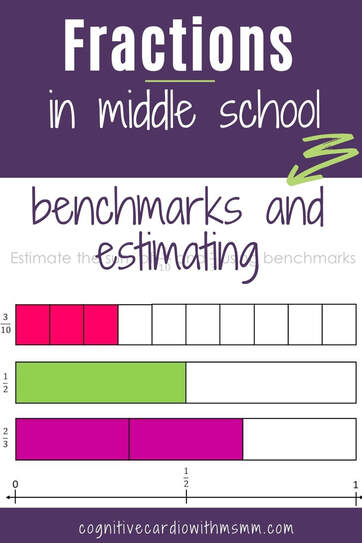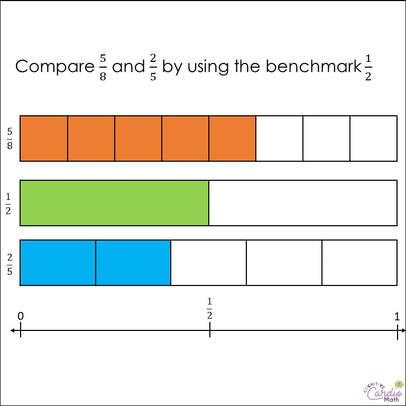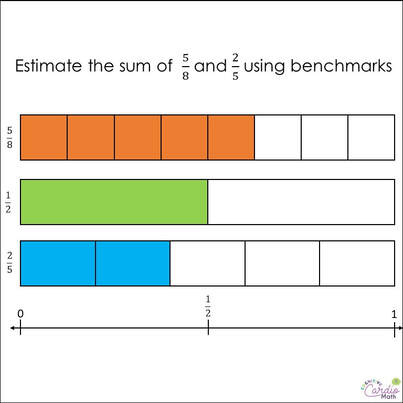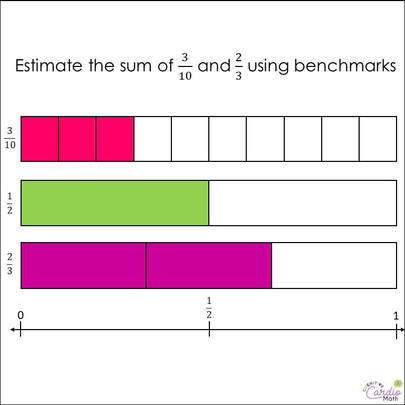# Teaching Fractions: Benchmarking and Estimation

## Fraction Series, Week 3: Benchmarks and EstimationIn the fraction standards review part of last week’s fraction post, I briefly summarized the fraction skills addressed at different grade levels.
In 4th grade, we have “comparing fractions with different numerators and denominators by using common denominators or benchmarks.”

When we move to the 5th grade standards, we see benchmarking is also included in 5.NF.A.2:

• “Solve word problems involving addition and subtraction of fractions referring to the same whole, including cases of unlike denominators, e.g., by using visual fraction models or equations to represent the problem. Use benchmark fractions and number sense of fractions to estimate mentally and assess the reasonableness of answers.”

## Using Fraction Benchmarks​Using benchmarks to estimate is a powerful way to help students understand whether or not their answers make sense.
I’m not sure if your experience is the same as mine, but over the years, I’ve observed that this understanding of whether or not answers make sense is definitely an area of weakness for students (not just in the area of fractions).
So, if we can incorporate the reinforcement of benchmarks, estimating, and the understanding of ‘reasonable’ it will be a huge benefit to our middle school students.

Let’s look at the 4th grade concept of using benchmarks to compare fractions.
When students are asked to compare fractions like 5/8 and 2/5, for example, the standard would like them to be able to look at 5/8 and think:

• Hmm, 4/8 is half (benchmark) and 5/8 is more than that
• 2.5/5 would be exactly half, and 2/5 is less than that
• So, if 5/8 is more than half and 2/5 is less than half, that means 5/8 must be greater than 2/5

​This is great logic. I have found, however, that some students don’t know how to find half of a number and even if they do know, they have trouble with half of an odd number. Have you found this too? That may be something for another post!

When it comes to using benchmarks in 5th and 6th grades and beyond, we might not be using them as much to teach comparing fractions; but we can teach using benchmarks (and finding half) in the context of estimating answers for adding and subtracting fractions (and eventually for multiplying and dividing).

## Fraction Benchmarks to Estimate Fraction Addition

Let’s look at how we might teach estimating a sum with benchmarks, using the same fractions we compared: 5/8 + 2/5
1) Before adding, ask students to estimate the answer by changing each fraction to a benchmark of 0, ½, or 1.
2) Estimating 5/8 prompts the question ‘how many 8ths would be in exactly half?’ (bulleted items are a sample discussion between teacher and student)

• Student: 4/8
• Teacher: How do you know?
• Student: because 8 divided by 2 is 4
• Teacher: That’s right, to find half of a number, we divide by 2. So, is 5/8 close to 4/8?
• Student: yes
• Teacher: Is 5/8 closer to 0,½, or 1?
• Student: ½

3) Same process for 2/5: how many 5ths would be in exactly ½?’

• Student: 2.5
• Teacher: How do you know?
• Student: because 5 divided by 2 is 2.5
• ​Teacher: is 2.5/5 close to 2/5?
• Student: yes
• Teacher: Is 2/5 closer to 0,½, or 1?
• Student: ½

4) Summarize estimation of the addends to get to estimation of the sum:

• If 5/8 is close to ½ and 2/5 is close to ½, about how much should the final answer be?
• Student: 1
• Teacher: Why?
• Student: because ½ and ½ = 1

## Include Models for Fraction Estimation​Along with these conversations, we can add some visual models.
If you have fraction strips to show these fractions quickly, that’s great. If not a quick drawing will help. (Personally, I might have some difficulty making these quickly and accurately, so I created a PDF download with the fraction strips to go with the examples in this post.)

If students are having difficulty with finding half of 8 and 5, a visual model like this will allow them to line up the
½ mark on the ½ strip with the 8ths and 5ths, and see exactly where the fraction falls. They can see more clearly how close the fractions are to 0, ½, and 1.

While estimation may cause the fraction addition or subtraction problems to take a little longer, the extra time and modeling reinforces several concepts: finding half, comparing fractions with benchmarks, and estimating to see if the answer is reasonable.

## Include Models for Fraction Estimation: Example 2One more example: 3/10 + 2/3

Again providing a visual model is very helpful.
In this case, the number line may be even more helpful than in the previous example.
These fractions ARE closer to ½, but not as close as those in the previous example:

• Students may think that 3/10 is closer to 0 than ½ if they can’t see it.
• Students may think 2/3 is closer to 1 than ½ if they can’t see it.

Reasoning for 3/10:

• Half of 10 is 5, so 5/10 is halfway
• 3 is closer to 5 than it is to 0, so ½ would be the better estimate.

Reasoning for 2/3:

• Half of 3 is 1.5
• 2 is closer to 1.5 than it is to 3, so again ½ is the closer benchmark

Do you use benchmarking and estimation on a regular basis when you’re working with fractions in your classroom?
Interested in more fraction and fraction operation content?
Check out the course,

## EllieWelcome to Cognitive Cardio Math! I’m Ellie, a wife, mom, grandma, and dog ‘mom,’ and I’ve spent just about my whole life in school! With nearly 30 years in education, I’ve taught:

• All subject areas in 4th and 5th grades
• Math, ELA, and science in 6th grade (middle school)

I’ve been creating resources for teachers since 2012 and have worked in the elearning industry for about five years as well!